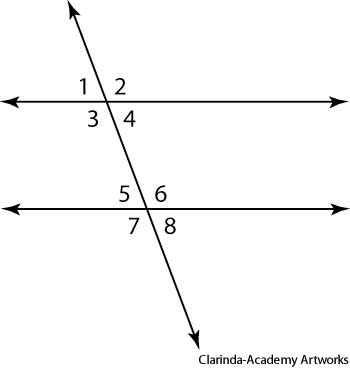# parallel line

what is parallel line

Parallel lines are lines in a plane which do not intersect. Like adjacent lanes on a straight highway, two parallel lines face in the same direction, continuing on and on and never meeting each other. In the figure in the first section below, the two lines AB↔\overleftrightarrow{AB} and CD↔\overleftrightarrow{CD} are parallel. The symbol for parallel lines is ∥,\parallel, so we can say that AB↔∥CD↔\overleftrightarrow{AB}\parallel\overleftrightarrow{CD}

in that figure.

According to the axioms of Euclidean geometry, a line is not parallel to itself, since it intersects itself infinitely often. However, some authors allow a line to be parallel to itself, so that “is parallel to” forms an equivalence relation.

what is interior angleInterior angle is any of the four angles formed between two straight lines intersected by a third straight line.